## Example Questions

### Example Question #1 : How To Find The Volume Of A Cube

What is the volume of a rectangular box that is twice as long as it is high, and four times as wide as it is long?

2L2

8

5L

2L3

4L3

2L3

Explanation:

The box is 2 times as long as it is high, so H = L/2. It is also 4 times as wide as it is long, so W = 4L. Now we need volume = L * W * H = L * 4L * L/2 = 2L3.

### Example Question #2 : Cubes

What is the volume of a cube with a surface area of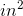?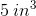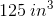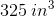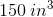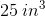Explanation:

The surface area of a cube is merely the sum of the surface areas of thesquares that make up its faces. Therefore, the surface area equation understandably is: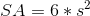, whereis the side length of any one side of the cube. For our values, we know: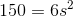Solving for, we get: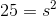or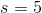Now, the volume of a cube is defined by the simple equation: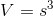For, this is: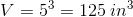### Example Question #10 : Cubes

The volume of a cube is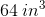. If the side length of this cube is tripled, what is the new volume?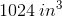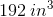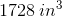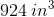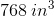Explanation:

Recall that the volume of a cube is defined by the equation:, whereis the side length of the cube.

Therefore, if we know that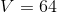, we can solve: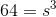This means that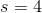.

Now, if we tripleto, the new volume of our cube will be: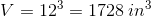### Example Question #331 : Geometry

What is the volume of a cube with surface area of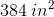?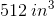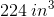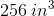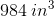Explanation:

Recall that the equation for the surface area of a cube is merely derived from the fact that the cube's faces are made up ofsquares. It is therefore: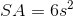For our values, this is: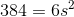Solving for, we get: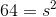, so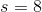Now, the volume of a cube is merely:Therefore, for, this value is: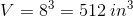### Example Question #332 : Geometry

A cube has a volume of 64, what would it be if you doubled its side lengths?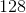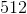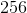Explanation:

To find the volume of a cube, you multiple your side length 3 times (s*s*s).

To find the side length from the volume, you find the cube root which gives you 4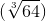.

Doubling the side gives you 8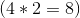.

The volume of the new cube would then be 512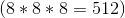.

Tired of practice problems?

Try live online GRE Math prep today.